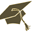Témata prací (Výběr práce)(verze: 363)
Detail práce
Přihlásit přes CASUmělé neuronové sítě jako náhradní modely v optimalizaci
Název práce v češtině: Umělé neuronové sítě jako náhradní modely v optimalizaci Artificial neural networks as surrogate models in optimization 2023/2024 disertační práce Katedra teoretické informatiky a matematické logiky (32-KTIML) prof. RNDr. Ing. Martin Holeňa, CSc.
 Zásady pro vypracování As black-box optimization, we refer to optimization in which the mathematical expression of the optimized function is not used (typically because no such expression is known), but the optimization algorithm only has its values at specific points available. These values are usually obtained empirically by measurement or through experiments, either physical or in the form of simulations. Black-box optimization uses algorithms that make almost no assumptions about the mathematical properties of the optimized function, most often evolutionary algorithms and other nature-inspired algorithms such as particle swarms. Since these algorithms work only with the functional values of the optimized function, they approach its optimum much more slowly than the optimization methods for smooth functions, which also use information about the gradient or second derivatives of the optimized function. This property is particularly disadvantageous in conjunction with the fact that empirically obtaining the value of the optimized function is usually quite expensive and time-consuming. However, evolutionary algorithms can be significantly accelerated by using the empirical black-box function only occasionally for evaluating the value of the optimized function, whereas mostly only its sufficiently accurate regression model is evaluated. Among the regression models used for this purpose, artificial neural networks have been for about 20 years, first multilayer perceptrons and later networks with radial basis functions. Due to the current popularity of modern types of neural networks, often referred to as deep neural networks, new approaches usable speed up black-box optimization based on modern neural networks have nevertheless been proposed in recent years, such as deep Gaussian processses, using Bayesian neural networks, optimization in a latent space of a lower dimension, mapped by a generative neural network into the spacein which the inputs of the optimized black-box function lie, or making use of GAN-type networks (generative adversarial network), the two components of which are used for the exploration and exploitation components of the optimization.
 Seznam odborné literatury Will be provided by the supervisor.